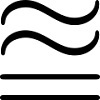# Resources tagged with: Inequalities

Filter by: Content type:
Age range:
Challenge level:

### There are 16 results

Broad Topics > Algebraic expressions, equations and formulae > Inequalities### Square Mean

##### Age 14 to 16Challenge Level

Is the mean of the squares of two numbers greater than, or less than, the square of their means?### Biggest Enclosure

##### Age 14 to 16Challenge Level

Three fences of different lengths form three sides of an enclosure. What arrangement maximises the area?##### Age 14 to 16Challenge Level

Kyle and his teacher disagree about his test score - who is right?### Proofs with Pictures

##### Age 14 to 18

Some diagrammatic 'proofs' of algebraic identities and inequalities.### ' Tis Whole

##### Age 14 to 18Challenge Level

Take a few whole numbers away from a triangle number. If you know the mean of the remaining numbers can you find the triangle number and which numbers were removed?### Not Continued Fractions

##### Age 14 to 18Challenge Level

Which rational numbers cannot be written in the form x + 1/(y + 1/z) where x, y and z are integers?### Two Cubes

##### Age 14 to 16Challenge Level

Two cubes, each with integral side lengths, have a combined volume equal to the total of the lengths of their edges. How big are the cubes? [If you find a result by 'trial and error' you'll need to. . . .### Rationals Between...

##### Age 14 to 16Challenge Level

What fractions can you find between the square roots of 65 and 67?### Unit Interval

##### Age 14 to 18Challenge Level

Take any two numbers between 0 and 1. Prove that the sum of the numbers is always less than one plus their product?### Fracmax

##### Age 14 to 16Challenge Level

Find the maximum value of 1/p + 1/q + 1/r where this sum is less than 1 and p, q, and r are positive integers.### All-variables Sudoku

##### Age 11 to 18Challenge Level

The challenge is to find the values of the variables if you are to solve this Sudoku.### Inequalities

##### Age 11 to 14Challenge Level

A bag contains 12 marbles. There are more red than green but green and blue together exceed the reds. The total of yellow and green marbles is more than the total of red and blue. How many of. . . .### Plutarch's Boxes

##### Age 11 to 14Challenge Level

According to Plutarch, the Greeks found all the rectangles with integer sides, whose areas are equal to their perimeters. Can you find them? What rectangular boxes, with integer sides, have. . . .### Almost Total Inequality

##### Age 14 to 16Challenge Level### Tet-trouble

##### Age 14 to 16Challenge Level

Is it possible to have a tetrahedron whose six edges have lengths 10, 20, 30, 40, 50 and 60 units?### Approximating Pi

##### Age 14 to 18Challenge Level

By inscribing a circle in a square and then a square in a circle find an approximation to pi. By using a hexagon, can you improve on the approximation?